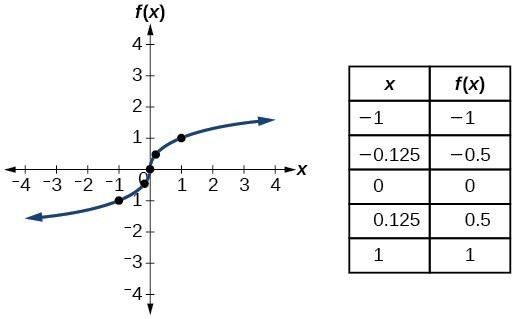# Identifying Basic Toolkit Functions

In this text, we will be exploring functions—the shapes of their graphs, their unique characteristics, their algebraic formulas, and how to solve problems with them. When learning to read, we start with the alphabet. When learning to do arithmetic, we start with numbers. When working with functions, it is similarly helpful to have a base set of building-block elements. We call these our “toolkit functions,” which form a set of basic named functions for which we know the graph, formula, and special properties. Some of these functions are programmed to individual buttons on many calculators. For these definitions we will use $x$ as the input variable and $y=f\left(x\right)$ as the output variable.

We will see these toolkit functions, combinations of toolkit functions, their graphs, and their transformations frequently throughout this book. It will be very helpful if we can recognize these toolkit functions and their features quickly by name, formula, graph, and basic table properties. The graphs and sample table values are included with each function shown below.

Toolkit Functions
Name Function Graph
Constant $f\left(x\right)=c$, where $c$ is a constant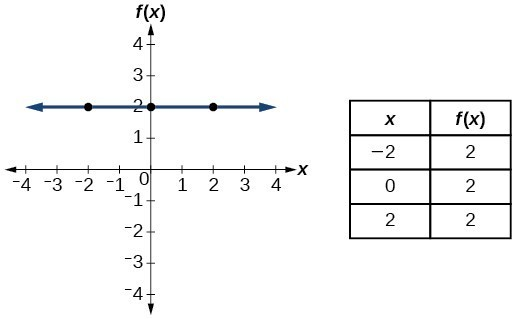Identity $f\left(x\right)=x$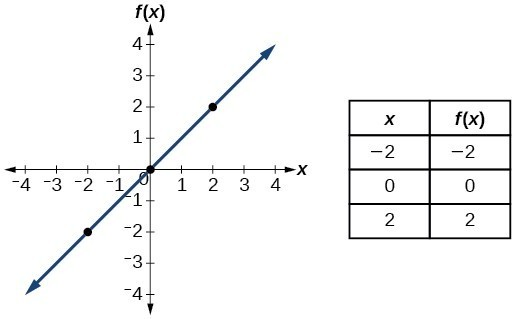Absolute value $f\left(x\right)=|x|$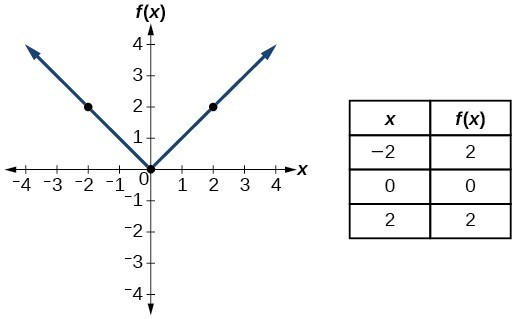Quadratic $f\left(x\right)={x}^{2}$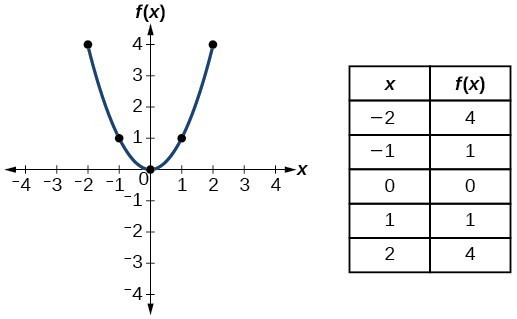Cubic $f\left(x\right)={x}^{3}$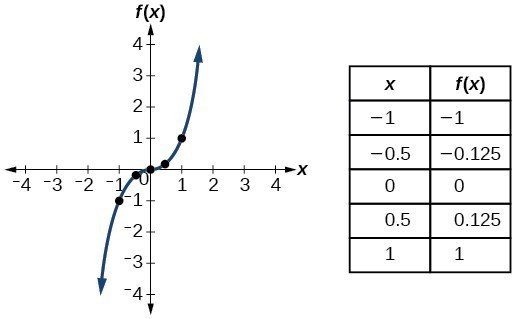Reciprocal $f\left(x\right)=\frac{1}{x}$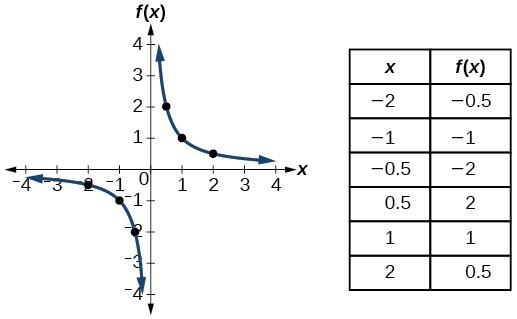Reciprocal squared $f\left(x\right)=\frac{1}{{x}^{2}}$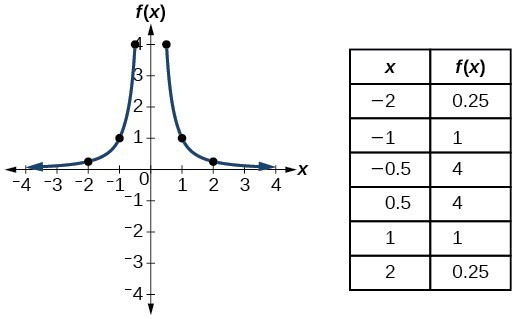Square root $f\left(x\right)=\sqrt{x}$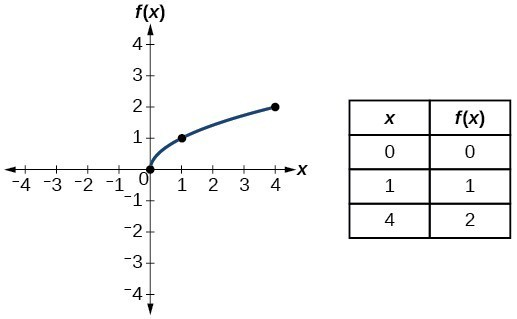Cube root $f\left(x\right)=\sqrt{x}$